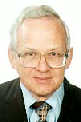Electron.n. J. Diff. Eqns., Vol. 1999(1999), No. 07, pp. 1-37.

### Distributional asymptotic expansions of spectral functions and of the associated Green kernels R. Estrada & S. A. Fulling

Abstract:
Asymptotic expansions of Green functions and spectral densities associated with partial differential operators are widely applied in quantum field theory and elsewhere. The mathematical properties of these expansions can be clarified and more precisely determined by means of tools from distribution theory and summability theory. (These are the same, insofar as recently the classic Cesaro-Riesz theory of summability of series and integrals has been given a distributional interpretation.) When applied to the spectral analysis of Green functions (which are then to be expanded as series in a parameter, usually the time),these methods show: (1) The ``local'' or ``global'' dependence of the expansion coefficients on the background geometry, etc., is determined by the regularity of the asymptotic expansion of the integrand at the origin (in ``frequency space''); this marks the difference between a heat kernel and a Wightman two-point function, for instance. (2) The behavior of the integrand at infinity determines whether the expansion of the Green function is genuinely asymptotic in the literal, pointwise sense, or is merely valid in a distributional (Cesaro-averaged) sense; this is the difference between the heat kernel and the Schrodinger kernel. (3) The high-frequency expansion of the spectral density itself is local in a distributional sense (but not pointwise). These observations make rigorous sense out of calculations in the physics literature that are sometimes dismissed as merely formal.

Submitted April 29, 1998. Published March 1, 1999.
Math Subject Classification: 35P20, 40G05, 81Q10.
Key Words: Riesz means, spectral asymptotics, heat kernel, distributions.Ricardo Estrada P. O. Box 276 Tres Rios, Costa Rica e-mail: restrada@cariari.ucr.ac.crS. A. Fulling Department of Mathematics Texas A&M University College Station, Texas 77843-3368 USA e-mail: fulling@math.tamu.edu